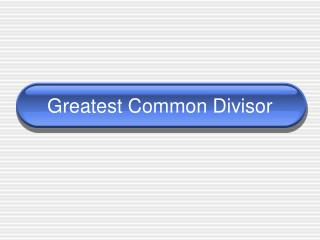DownloadDownload PresentationGreatest Common Divisor

# Greatest Common Divisor

Download Presentation## Greatest Common Divisor

- - - - - - - - - - - - - - - - - - - - - - - - - - - E N D - - - - - - - - - - - - - - - - - - - - - - - - - - -
##### Presentation Transcript

1. Greatest Common Divisor

2. Definitions • Let a and b be two non-zero integers. The greatest common divisor of a and b, denoted gcd(a,b) is the largest of all common divisors of a and b. • When gcd(a,b) = 1, we say that a and b are relatively prime.

3. Examples • gcd(10,25) = • gcd(10,26) = • gcd(10,27) = so 10 and 27 are relatively prime! • gcd(10,30) = • gcd(-10,-30) = • gcd(13978,15457) = 5 2 1 10 10 29

4. Thm 0.2 GCD is a Linear Combination • For any nonzero integers a and b, there exist integers s and t such that gcd(a,b) = as + bt • Moreover, gcd(a,b) is the smallest postive integer of the form as + bt. • Note: This is the most important thing to know about greatest common divisors!

5. Example • gcd(32,14) = 2 • Check that 32(-3) + 14(7) = 2 • Also, 32(11) + 14(-25) = 2 • In fact, there are infinitely many ways to write 2 as a linear combination. • 2 is the smallest postive linear combination of 32 and 14. • (Also, 2 | 32s + 14t for all integers s, t)

6. Example • gcd(13978,15457) = 29 • Check that 13978(209) + 15457(-189) = 29 • Also, 13978(-15248) + 15457(13789) = 29 • 29 is the smallest postive linear combination of 13978 and 15457.

7. Proof • The proof consists of three steps. • Show how to choose an integer d > 0 that could be the gcd of a and b. • Show that d is a common divisor, I.e. show that d | a and d | b • Show that any other common divisor c of a and b must also divide d.

8. 1. Choose d • Given non-zero integers a,b, let S be the set of positive linear combinations of a,b. S = {as+bt | s,t are integers and as+bt > 0} • Clearly S is a set of non-empty positive integers. By the WOP, S has a least element, d.

9. 2: Show d|a and d|b • Divide a by d, recalling that d = as + bt. a =dq + r = (as + bt)q + r, where 0 ≤ r < d. Then r = a(1-sq)+b(-tq) So r isa linear combination of a and b. • If r > 0, then r would have to be in S. But this is impossible, since r < d. So r must be 0, a = dq, and d|a. • Use symmetry (exchange the names of a and b) to see that d|b as well as a. • So d is a common divisor, as required.

10. 3. Show any other common divisor must divide d • Let c be any common divisor of a and b. Say a = ch and b = ck. • Then d = as + bt = chs + ckt = c(hs+kt) • So c|d.

11. Corollary • If a and b are relatively prime, then there are integers s and t with as + bt = 1.

12. || || || || || || X X X X X X + + + + + + gcd = Euclidean Algorithm 15457 + 209 13978 1 – 189 1479 9 + 20 667 2 – 9 145 4 + 2 87 1 – 1 58 1 + 1 29 2 0 15457(-189)+13978(209)=29 0